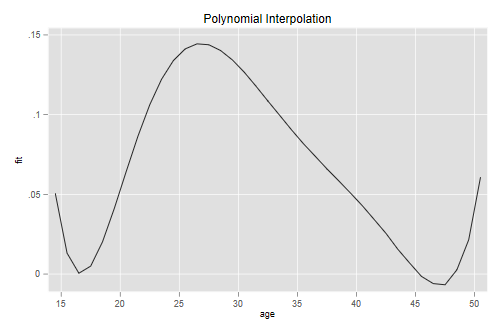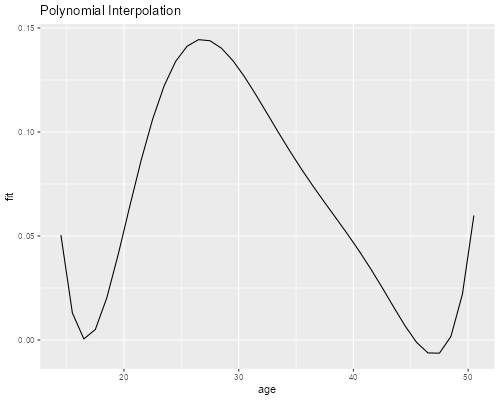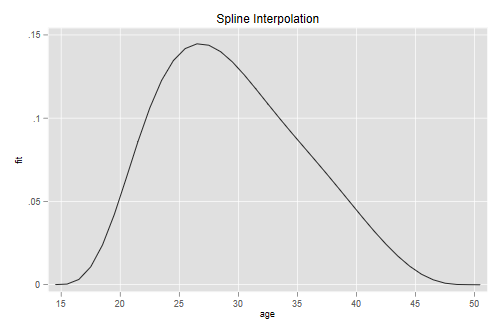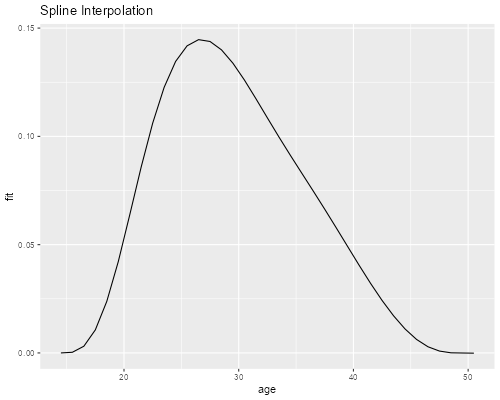Germán Rodríguez
Demographic Methods Princeton University## Interpolation

Splines can also be used for interpolation. Here we reproduce the results in the article by Mc Neil, Trussell and Turner listed in the readings. Their objective is to produce single-year fertility rates by interpolating in a five-year schedule. This log is fairly technical and you may wish to skip it unless you have an interpolation problem at hand. The gist is that polynomials can be troublesome while splines are better behaved.

### The Data

The data represent cumulative fertility at ages 15(5)50, which we will just type in

```. clear

. mata: mtt = range(15,50,5), (0\ 0.080\ 0.593\ 1.297\ 1.840\ 2.171\ 2.296\ 2.30
> 6)

. getmata (age F) = mtt
```
```> mtt <- data.frame(age = seq(15, 50, 5),
+   F = c(0, 0.080, 0.593, 1.297, 1.840, 2.171, 2.296, 2.306))
```

### Polynomial Interpolation

With 8 data points we can get an exact fit using a 7-th degree polynomial. Let us reproduce Figure 1 in the article, showing that polynomials don't work very well in this case. We need age^2 to age^7.R can build orthogonal polynomials for us.Because the fit is exact the residual sum of squares is 0 and the standard errors are undefined, so we will not print the results.

```. forvalues p=2/7 {
2.   gen age`p' = age^`p'
3. }

. quietly regress F age age2-age7
```
```> pf <- lm(F ~ poly(age, 7), data=mtt)
```

To do the interpolation we predict on a new dataset representing exact ages 14 to 51 (or try a slightly wider range to see how much worse it gets :)

```. drop _all

. set obs 38
Number of observations (_N) was 0, now 38.

. gen age = 13 + _n

. forvalues p=2/7 {
2.   gen age`p' = age^`p'
3. }

. predict Fit
(option xb assumed; fitted values)
```
```> library(dplyr)
> df <- data.frame(age = 14:51)
> df <- mutate(df, Fit = predict(pf, newdata=df))
```

Now that we have cumulative fertility at every age between 14 and 51 we can difference to obtain age-specific fertility rates centered at the midpoints of each year of age

```. gen fit = Fit - Fit[_n-1]
(1 missing value generated)

. gen agem = (age + age[_n-1])/2
(1 missing value generated)

. line fit agem, xtitle(age) xlabel(15(5)50) title(Polynomial Interpolation)

. graph export iasfrp.png, width(500) replace
file iasfrp.png saved as PNG format
``````> library(ggplot2)
> dfd <- data.frame( age = df\$age[-1] - 0.5, fit = diff(df\$Fit))
> ggplot(dfd, aes(age, fit)) + geom_line() + ggtitle("Polynomial Interpolation")
> ggsave("iasfrpr.png", width=500/72, height=400/72, dpi=72)
```Obviously the polynomial is not well behaved at the extremes. This type of result is not unusual with polynomials.

### Restricted Splines

McNeil et al. use a spline of degree five with internal knots at ages 20(5)45 and restricted to have zero first and second derivatives at ages 15 and 50 in order to ensure good behavior in the tails. The 5th degree polynomial has 6 parameters, the knots add 6, and the restrictions subtract 4, for a total of 8; exactly the same as the polynomial.

The article shows how to set up a system of linear equations to compute the coefficients, which can also be done via regression. To check our results against the paper we will rescale age so we work with 0(1)7 instead of 15(5)50.

This time the data will have 12 rows, 8 for cumulative fertility and four for the constraints

```. clear

. mata: mttx = (mtt[,1]\15\50\15\50), (mtt[,2]\J(4,1,0))

. getmata (age F ) = mttx
```
```> mttx <- rbind(mtt, data.frame(age = c(15, 50, 15, 50), F = rep(0, 4)))
```

Next we create the terms involving the powers and knots

```. gen one = 1

. replace one = 0 in 9/12

. // powers
. gen a = (age - 15)/5

. forvalues p=2/5 {
2.   gen a`p' = a^`p'
3.   quietly replace a`p' = `p' *  a^(`p' - 1) in 9/10
4.   // 1st der
.   quietly replace a`p' = `p' * (`p' - 1) * a^(`p' - 2) in 11/12
5.   // 2nd der
. }

. // knots
. forvalues i=1/6 {
2.   gen knot`i' =             cond(a > `i',    (a - `i')^5, 0)
3.   quietly replace knot`i' = cond(a > `i',  5*(a - `i')^4, 0) in  9/10
4.   // 1st
.   quietly replace knot`i' = cond(a > `i', 20*(a - `i')^3, 0) in 11/12
5.   // 2nd
. }

. replace a = 1 in  9/10

. replace a = 0 in 11/12
```
```> d0 <- 1:8; d1 <- 9:10; d2 <- 11:12 # location of data and constraints
> mttx[d0,       "one"] <- 1
> mttx[c(d1,d2), "one"] <- 0
> # powers
> a <- (mttx\$age - 15)/5 # for short
> for(p in 2:5) {
+   ap <- paste("a", p, sep="")
+   mttx[d0, ap] <- a[d0]^p
+   mttx[d1, ap] <- p * a[d1]^(p-1)
+   mttx[d2, ap] <- p * (p-1) * a[d2]^(p-2)
+ }
> # knots
> for(i in 1:6) {
+   ki <- paste("k", i, sep="")
+   mttx[d0, ki] <- ifelse(a[d0] > i,    (a[d0] - i)^5, 0)
+   mttx[d1, ki] <- ifelse(a[d1] > i,  5*(a[d1] - i)^4, 0)
+   mttx[d2, ki] <- ifelse(a[d2] > i, 20*(a[d2] - i)^3, 0)
+ }
> mttx[   ,"a"] <- a
> mttx[d1, "a"] <- 1
> mttx[d2, "a"] <- 0
```

You should probably list the data to have a look at the structure. To get the coefficients we run a regression. The fit is perfect so we suppress detailed results.we use `solve()`, but could use `lm()` as well.

```. quietly regress F one a a2-a5 knot*, noconstant

. set linesize 80

. mat list e(b)

e(b)[1,12]
one           a          a2          a3          a4          a5
y1   1.853e-10   2.042e-10   4.158e-11   .02094163   .09502961  -.03597124

knot1       knot2       knot3       knot4       knot5       knot6
y1   .05607291  -.01914399  -.00640362   .00816153  -.00289059  -.00586751
```
```> X <- mttx[,c(3,14,4:13)]  # one, a, a2-a5, k1-k6
> b <- solve(X, mttx\$F); b
one             a            a2            a3            a4
0.000000e+00  4.324583e-15 -1.694940e-14  2.094161e-02  9.502964e-02
a5            k1            k2            k3            k4
-3.597125e-02  5.607293e-02 -1.914401e-02 -6.403600e-03  8.161524e-03
k5            k6
-2.890595e-03 -5.867494e-03
```

The coefficients are exactly the same as in the paper (see page 252), with the first three rounded to zero. Next we need a prediction dataset in single years, with the power and knot terms but not the derivatives.

```. drop _all

. set obs 38
Number of observations (_N) was 0, now 38.

. gen one = 1

. gen age = 13 + _n

. gen a = (age - 15)/5

. forvalues p=2/5 {
2.   gen a`p' = a^`p'
3. }

. forvalues i=1/6 {
2.   gen knot`i' = cond(a > `i', (a - `i')^5, 0)
3. }

. predict Fit
(option xb assumed; fitted values)
```
```> nd <- data.frame(age = 14:51) |> mutate(one = 1, a = (age-15)/5)
> for (p in 2:5) {
+   ap = paste("a", p, sep="")
+   nd[, ap] = nd\$a^p
+ }
> for (i in 1:6) {
+   ki <- paste("k", i, sep="")
+   nd[, ki] <- ifelse(nd\$a > i, (nd\$a - i)^5, 0)
+ }
> Fit = as.matrix(nd[, -1]) %*% b
```

Which we then difference and plot

```. gen fit = Fit - Fit[_n-1]
(1 missing value generated)

. gen agem = (age + age[_n-1])/2
(1 missing value generated)

. line fit agem, xtitle(age) xlabel(15(5)50) title(Spline Interpolation)

. graph export iasfrs.png, width(500) replace
file iasfrs.png saved as PNG format
``````> nd = data.frame(age = 14:50 + 0.5, fit = diff(Fit))
> ggplot(nd, aes(age, fit)) + geom_line() + ggtitle("Spline Interpolation")
> ggsave("iasfrsr.png", width=500/72, height=400/72, dpi=72)
```Obviously the restricted spline is much better behaved at the extremes!

